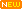Study specialized technical articles, papers & courses in low/high voltage fields. Save 50% on all courses at the EEP Academy with the Enterprise Plan.

# Spreadsheet for Transformer Losses Calculation

## Transformer Losses

Transformers have two major components that drive losses: the core and the coils. The typical core is an assembly of laminated steel, and core losses are mostly related to magnetizing (energizing) the core.

These losses, also known as no-load losses, are present all the time the transformer is powered on – regardless of whether there is any load or not.

Core losses are roughly constant from no-load to full-load when feeding linear loads. They represent a continuous cost, 24 hours/day, for the 25-year or more life of the transformer.

• HV Full load current = VA / (1.732 · Volt)
• LV Full load current = VA / (1.732 · Volt)
• HV Side I2R losses = I²R · 1.5
• LV Side I²R losses = I²R · 0.5 · 3
• Total I² R lossses at Amb. temp = Hv losses + Lv losses
• Total Stray losses at Amb. temp = Measured losses – I²R losses
• I²R lossses at 75° C temp = ((225 + 75) · losses) / (225 + Amb. temp) .
• Stray losses at 75° C temp=((225 + Amb. temp)(Stray losses at Amb. temp)) / 300
• Total Full load losses at 75° C = I²R losses at 75° C + Stray losses at 75° C
• Total Impedance at amb. temp = (Imp. voltage · 1.732) / Full load current
• Total Resistance at amb. temp = I²R losses / I²
• Total Reactance (X) = SQRT (Impedance² – Resistance²)
• Resistance at 75° C = (300 · resistance at amb ) / (225 + Amb. temp)
• Impedance at 75° C = SQRT (R² at 75° C + X²)
• Percentage Impedance = (Z at 75° C · I · 100)/V1
• Percentage Resistance = ( R 75° C · I · 100)/V1
• Percentage Reactance = (X · I · 100) / V
• Regulation at Unity P.F. = (%R cosø + %Xsinø)
• Regulation at 0.8 P.F. = (%R cosø + %Xsinø) + 1/200(%R sinø – %Xcosø)2

#### Efficiency at Unity P.F

• At 125 % of Transformer Loading = (kVA · 1.25 · 100)/((kVA · 1.25)+(I²R losses · 1.25²)+(No Load Losses))

#### Efficiency at 0.8 P.F

• At 125 % of Transformer Loading = (kVA · 1.25 · P.F. · 100)/((kVA · P.F. · 1.25)+(I²R losses · 1.25²)+(No Load Losses))

## Contribution to transformer losses

This difference substantially impacts actual losses incurred. Unfortunately, there is a small body of field data available, driven by the factors such as a lack of awareness of the cost of the losses, and the cost of gathering detailed data from a reasonable number of individual transformers.

Several variables contribute to transformer losses, the most important of which include load level, load profile, and core and coil construction.

Since there are a wide variety of transformers on the market serving different purposes, and available from different manufacturers, actual losses incurred in the field will vary substantially from installation to installation.

 Software: Spreadsheet for Transformer Losses Calculation Version: 10.06.2013Developer: Jignesh Parmar Size: 36 Kb Price: Free Download: Right here | Video Courses | Membership | Download Updates

1.Denis
Jun 16, 2022

Dear Jignesh,
I don’t find Lv resistance nor Hv resistance (cells C16 and C17 in your Excel file) on my transformer user manual. I though at first that it was primary side resistance and secondary side resistance. But in that case, I don’t understand why you multiply by 0.5 in the I²R losses formula (cells H7 and H8).
I thought it might be total resistance seen from primary and secondary, thus I computed I²R losses / I1² and the same with I2², but figures are different.

Please, can you explain me how to find these values (Lv and Hv resistance) ?

With kind regards
Denis

2.Pankaj Patel
Dec 30, 2021

Dear sir,
How to calculate 2500KVA (11kv/415V) Transformer no load loss and copper loss at 50% rated load.
Transformer details:
Make- Crompton
YOM- 2007
KVA- 2500
Impedance volts- 7.10%
HV Amp- 131
LV Amp- 3333
Volts HV- 11000
Volts LV- 433
Type of cooling- ONAN

Regards,
Pankaj Patel
9879735195
Gujarat, India

•Wasiullah
Apr 21, 2022

Dear Mr. Pankaj patel,

This is very simple to measure no load losses.
First of all Switch off the transformer output breaker, which is installed on LT side called ACB. Then take Clamp meter and measure the Amps from transformer input (HT side) and put the amps in power formula, (P=HT Volt*HT Amps*pf*1.732/1000) P=11000*0.5*0.8*1.732/1000

Regards

3.Andrew Smith
Oct 05, 2021

Dear Sir,

Thank you for posting this calculator. I used it to check my own which was almost correct.

4.M.KISHORE BABU
Aug 06, 2021

Sir, please note that we have old 400 kva tranformer,year of manufactured 2007 and MAKE: CROMPTON GREAVES. OIL COOLED TRANFORMER Hence kindly suggest us for replacement of the TRANSFORMER , since it is very old.we are assuming that lot of energy getting wasted due to the above conditions.

•Esennar Transformers
Sep 14, 2021

You can go with Esennar Transformers.. where you can save upto 50 thousands rupees per year..

5.Mohd Zulhizam
Mar 11, 2021

Assuming the losses and impedance of the transformer is negligible, calculate the HV and LV currents if the transformer was loaded at 120%

What formula use ?

6.Shafique
Jun 26, 2020

Sir, coul you explain temperature gradient calculation of HV and LV winding , if temperature rise 40/50

7.Rohit Saini
Dec 12, 2019

8.Nandini Basavarajappa Doddaveerappla
Oct 15, 2019

Tell me how to get transformer copper loss
I have no load losses n total power loss

•vivek kumar
Jan 08, 2021

Total Power loss = No load loss + Copper loss + Stray loss

If assume Stray loss negligible then

Copper loss = Total power loss – No load loss

9.RAJESHKUMAR
Aug 28, 2019

Dear sir,
Dry type and oil type transformer losses how to calculate.

10.FLOYD
Jan 19, 2019

specs of hi pot tester from 10 to 35kv?